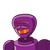# The Digit in the tens place of a 2 digit number is 3 more than the Digit in the units place. Let the Digit at units place be B. Th

The Digit in the tens place of a 2 digit number is 3 more than the Digit in the units place. Let the Digit at units place be B. Then the number is?​

### 1 thought on “The Digit in the tens place of a 2 digit number is 3 more than the Digit in the units place. Let the Digit at units place be B. Th”

1.